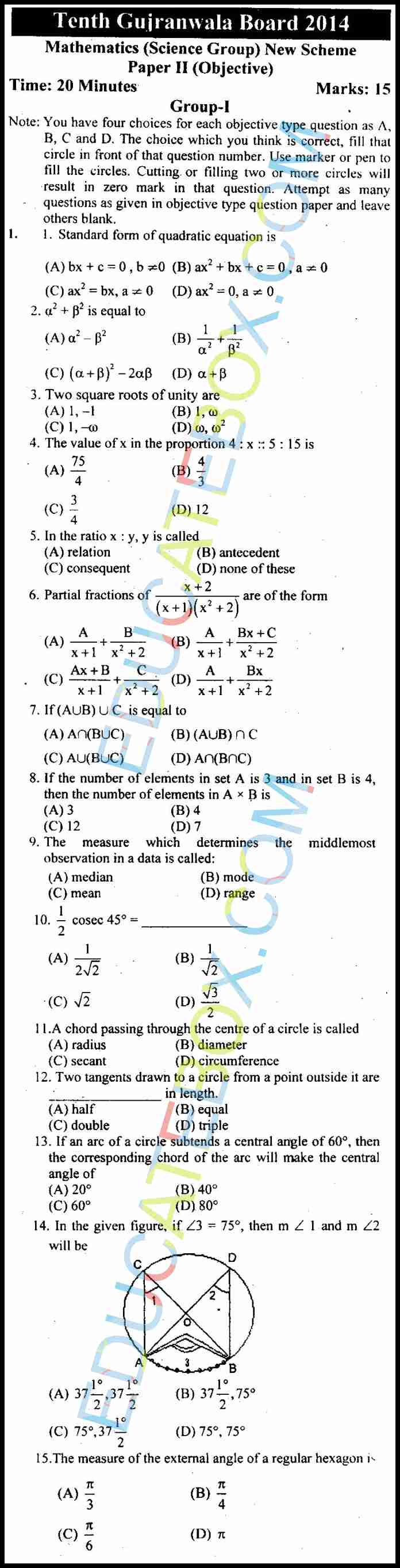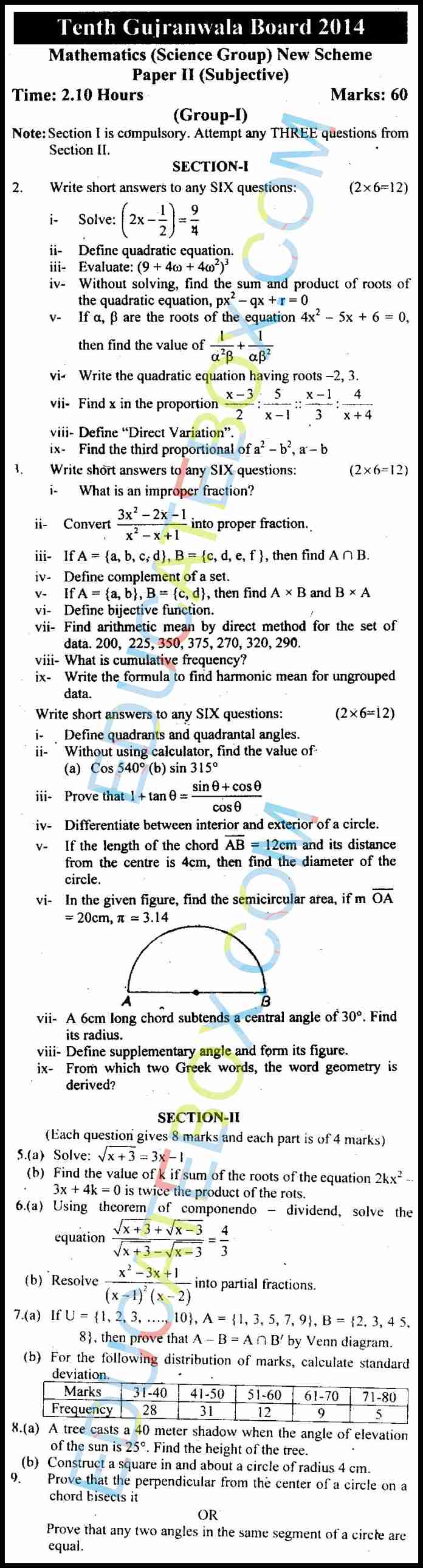# Past Paper 10th Maths (Science Group) Gujranwala 2014 Group1 (English Medium)

0
157

## Past Paper Class 10 Maths (Science Group) Gujranwala Board 2014 OBJECTIVE Type Group 1 (English Medium)

Maths (Science Group) 10th class 2014 paper of Gujranwala group 1 is a combination of one objective question and eight subjective questions. The objective question consists of 15 multiple choice questions, while subjection question paper consists of short questions and long questions.  Basic concepts discussed in this paper include the standard form of quadratic equation, square roots of unity, proportion, ratio, partial fractions, unions of sets, drawing of circle. quadratic equation, product of roots of quadratic equation, direct variation, improper fraction, complement of a set, bijective function, arithmetic mean by direct method, cumulative frequency, formula to find harmonic mean for ungrouped data, quadrants and quadrantile angles, interior and exterior of circle, calculation of standard deviation,

You may notice that most of the questions in this paper are from Geometry section.## Past Paper Class 10 Maths (Science Group) Gujranwala Board 2014 SUBJECTIVE Type Group 1 (English Medium)